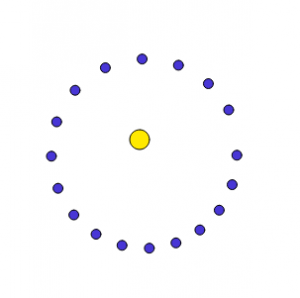# The Loop

In my previous article I described how one can use a differential equation to calculate the trajectory of the Earth around the Sun. But at the end it was quite tedious so I decided to use a computer instead. Because they are good at repeating things. One way to make a computer do the same things again and again is to use a loop. In the program below we will show how a for loop works:

```//position
var x = 100;
var y = 0;
var xp = 0;
var yp = 30;
var G = 1;
var m = 100000;

for (var i = 0; i < 18; i+=1) {
var d = Math.sqrt(xx + yy);
var a = Gm/(dd);
var xpp = -x/da;
var ypp = -y/da;
xp = xp + xpp;
yp = yp + ypp;
y = y + yp;
x = x + xp;

```fill(72, 54, 214);
ellipse(x + 200, - y + 200, 10, 10);
```

}
fill(255, 234, 0);
ellipse(200,200,20,20);
```
What the program does is to draw a planet 18 times using a for loop.In each iteration of the loop first of all you calculate the distance from the sun to the planet, after you need to calculate the acceleration then you should find the speed of the planet at the end you can find the position of the planet.

This video explains about the code above: